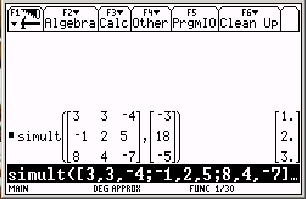# Knowledge Base

## Solution 12158: Solving System of Equations Using TI-89 Family, TI-92 Family and Voyage™ 200 Graphing Calculators.

### How do I solve system of equations on a TI-89 family, TI-92 family and Voyage 200 graphing calculator?

The example below will demonstrate how to use the SIMULT( function to solve system of equations on the TI-89 family, TI-92 family and Voyage 200.

For Example: Solve the following for x, y and z.

3x + 3y - 4z = -3
-x + 2y + 5z = 18
8x + 4y - 7z = -5

Solution:

1) Press [2ND] [MATH] to access the Math worksheet
2) Scroll down to #4 for Matrix and press the right arrow key
3) Scroll down to #5 and press [ENTER] to paste simult( on the command line
4) The syntax would then be:

simult ([3, 3, -4; -1, 2, 5; 8, 4, -7],[-3; 18; -5])

Please Note: Two matrices are actually entered: a three-by-three matrix of coefficients, and a one-by-three matrix of constants. Note that commas are used to separate entries within a matrix row and semicolons to separate the matrix rows themselves.

The TI calculator solves the system, and displays the system and the solution as follows:The answer is therefore, x=1, y=2, and z=3.

Solution using the SOLVE( function:

1) Press [F2] to access the Algebra worksheet
2) Press [ENTER] on 1:solve
3) Enter the function as listed below:

solve(3x+3y-4z=-3 and -x+2y+5z=18 and 8x+4y-7z=-5,{x,y,z}) and press [ENTER]

Please Note: Press [CATALOG] to access the "and" command or you can manually type "and" but you will need to enter a space before and after "and".

The answer is therefore x=1, y=2, z=3.

Please see the TI-89 Titanium / Voyage 200 guidebooks for additional information.